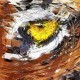# Drawing Circular Segments in Actionscript

This problem, in a couple different variations, has been presented to me a few times over the past two months so I thought it might be worth a blog post.  The problem ranges from drawing an aribtrary circular segment to the more general fractional circular area problem.  Since the former is covered in the latter, the problem discussed here is how to dynamically draw a portion of a circle that represents some fraction of the circle’s area.  Refer to the following diagram.The green shaded area represents a segment of the circle with radius r.  The general problem is given some multiplier, α in [0,1] how do we draw the green region so that its area is αAc, where Ac is the area of the circle. If α is less than or equal to 1/2, the problem reduces to drawing a circular segment at or below the horizontal axis passing through the center. For larger multipliers, we can locate the A and B points corresponding to a segment from the ‘top’ of the circle at a multiplier 1- α , drawing the complement of that segment.

Once the points A and B are identified, the outline of the circle is drawn with a sequence of quadratic Beziers just as in a wedge-drawing program with a line from A to B to complete the drawing. The quad. Bezier code for this example was extracted from my Singularity Wedge class.

To start the Bezier drawing, we need a start and end angle. Given the central angle of the sector, Θ, the start and end angles follow immediately.  You can look up the area of the segment on the web and equate it to a multiplier of the circle’s area.  This yields the equation

Θ – sin Θ = 2∏a .

This is not directly solvable for the central angle.  If the constant term on the right is moved to the left side of the equation, then the problem reduces to finding a zero of a function.  Ah, a numerical analysis problem … just what I like 🙂  I like it even more that I’m about to move and was organizing some folders containing old NA notes and had some scribbling on a good starting value for Θ – sin Θ, namely the cubed root of the constant term with a small multiplier.  So, Newton’s method should converge pretty quickly.

A standard Netwon iteration is used with f(Θ) = Θ – sin Θ – 2∏a and f'(Θ) = 1 – cos Θ .  The computation of start and end angles corresponding to the A and B points as well as the quad. Bezier code to trace the relevant circle outline can be deconstructed from the example code.

The following diagram shows an example of a multiplier greater than 1/2.  In the demo code, the slider is moved to vary the multiplier from 0 to 1.The code is all AS in Flash CS4.  No classes, just straight code on the timeline.  You can modify the code, repackage it into classes, do whatever you like.  Deconstruct and enjoy 🙂

As an aside, there is another approach to this problem that I like even better, but does not have the simple intro. to Newton’s method.  If time allows, I’ll blog about that one later.

good luck!

## 3 thoughts on “Drawing Circular Segments in Actionscript”

1.Mark says:

Wow awesome math!

(But why don’t you just use a square in a circulair mask that moves up/down?)

1.algorithmist says:

The original problem asked of me was how to do the drawing from scratch. If you want to move a mask up and down, you still need to figure out the distance from the center, which is a numerical problem just like determining the central angle in the sector.